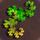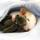## General Question# Help with math problem involving roots of a modulo operation?

Asked by PhiNotPi (12681) December 7th, 2010

I am trying to prove that the roots of the function y=[N (mod x)]+[N (mod N/x)] are all factors of N. I have already proved it by example.
Note: This is not a homework question. I am not in this level of math yet.

Observing members: 0Composing members: 0Question: if x doesn’t divide N, what does “N (mod N/x)” mean?

ratboy (15167)“Great Answer” (0) Flag as…@ratboy As an example of what it means, image a clock with 11.5 hours on it. It skips from eleven to zero faster than it should normally. Hours 1 – 11 will remain unchanged in mod 11.5, but 12 would cycle around and become 12 – 11.5 = 0.5. 50 will become 50 – 11.5 – 11.5 – 11.5 – 11.5 = 4.

PhiNotPi (12681)“Great Answer” (0) Flag as…Usually n (mod m) is considered non-negative, so that the sum could be 0 only if each of the summands were 0. Specifically: for integers n and m, n (mod m) = r iff for n = qm + r for some q and r where 0 <= r < N. It’s not trivial to extend the definition to real numbers since given any two non-zero real numbers, each divides the other evenly.

ratboy (15167)“Great Answer” (0) Flag as…or